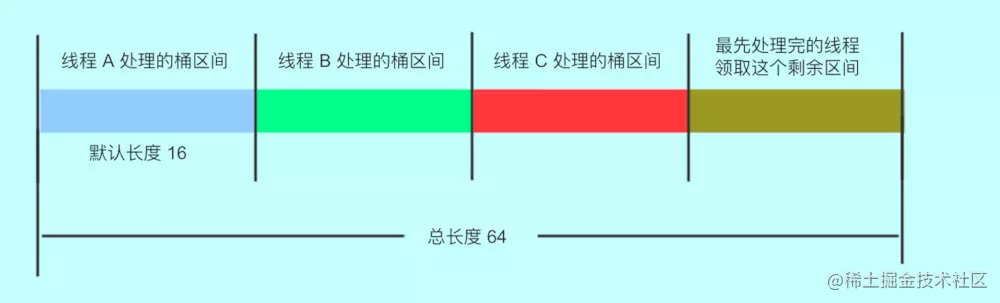# 面试：为了进阿里，死磕了ConcurrentHashMap源码和面试题(二)

• ConcurrentHashMap的实现原理
• ConcurrentHashMap1.7和1.8的区别？
• ConcurrentHashMap使用什么技术来保证线程安全
• ConcurrentHashMap的put()方法
• ConcurrentHashmap 不支持 key 或者 value 为 null 的原因？
• put()方法如何实现线程安全呢？
• ConcurrentHashMap扩容机制
• ConcurrentHashMap的get方法是否要加锁，为什么？
• 其他问题
• 为什么使用ConcurrentHashMap
• ConcurrentHashMap迭代器是强一致性还是弱一致性？HashMap呢？
• JDK1.7与JDK1.8中ConcurrentHashMap的区别

### ConcurrentHashMap的扩容机制

1. 扩容变量

``````// 新 tab 的 length
int nextn = nextTab.length;
// 创建一个 fwd 节点，用于占位。当别的线程发现这个槽位中是 fwd 类型的节点，则跳过这个节点。
ForwardingNode<K,V> fwd = new ForwardingNode<K,V>(nextTab);
// 首次推进为 true，如果等于 true，说明需要再次推进一个下标（i--）
//反之，如果是 false，那么就不能推进下标，需要将当前的下标处理完毕才能继续推进
// 完成状态，如果是 true，就结束此方法。
boolean finishing = false; // to ensure sweep before committing nextTab
复制代码``````
2. 因为`ConcurrentHashMap`支持多线程扩容，多个线程处理不同的节点，首先先计算出每个线程（CPU）处理的桶数：将 length / 8 然后除以 CPU核心数。如果得到的结果小于 16，那么就使用 16。（避免出现转移任务不均匀的现象）

``````int n = tab.length, stride;
if ((stride = (NCPU > 1) ? (n >>> 3) / NCPU : n) < MIN_TRANSFER_STRIDE)
stride = MIN_TRANSFER_STRIDE;
复制代码``````
3. 新的 table 尚未初始化，进行2倍扩容

`````` if (nextTab == null) {            // initiating
try {
// 扩容  2 倍
Node<K,V>[] nt = (Node<K,V>\[])new Node<?,?>[n << 1];
// 更新
nextTab = nt;
} catch (Throwable ex) {      // try to cope with OOME
// 扩容失败， sizeCtl 使用 int 最大值。
sizeCtl = Integer.MAX_VALUE;
return;// 结束
}
// 更新成员变量
nextTable = nextTab;
// 更新转移下标，就是 老的 tab 的 length
transferIndex = n;
}
复制代码``````
4. 在死循环中，每个线程先取得自己需要转移的桶的区间：先获取CAS 修改 transferIndex，即 length - 区间值，留下剩余的区间值供后面的线程使用(i 表示下标，bound 表示当前线程可以处理的当前桶区间最小下标)。

• 判断`--i`是否大于等于`bound` ，正常情况下，如果大于 bound 不成立，说明该线程上次领取的任务已经完成了。那么，需要在下面继续领取任务。
• `transferIndex` 小于等于0，说明没有区间了 ，i 改成 -1，推进状态变成 false，不再推进，表示，扩容结束了，当前线程可以退出了
• 第一次进入循环，走下面的 nextIndex 赋值操作（获取最新的转移下标）。其余情况都是：如果可以推进，将 i 减一，然后修改成不可推进。如果 i 对应的桶处理成功了，改成可以推进。
``````int nextIndex, nextBound;
if (--i >= bound || finishing)
//是为了防止在没有成功处理一个桶的情况下却进行了推进
else if ((nextIndex = transferIndex) <= 0) {
i = -1;
} else if ((nextIndex = transferIndex) <= 0) {
// 如果小于等于0，说明没有区间了 ，i 改成 -1，
//推进状态变成 false，不再推进，表示，扩容结束了，当前线程可以退出了
// 这个 -1 会在下面的 if 块里判断，从而进入完成状态判断
i = -1;
}
else if (U.compareAndSwapInt
(this, TRANSFERINDEX, nextIndex,
nextBound = (nextIndex > stride ?
nextIndex - stride : 0))) {
//当前线程可以处理的最小当前区间最小下标
bound = nextBound;
//初次对i 赋值，这个就是当前线程可以处理的当前区间的最大下标
i = nextIndex - 1;
}
复制代码``````
5. 判断该节点是否需要进行扩容处理

• 是否已完成扩容

• `finishing``true`，完成扩容
• 如果没完成
• 这个线程结束帮助扩容了`U.compareAndSwapInt(this, SIZECTL, sc = sizeCtl, sc - 1)`为true
• `(f = tabAt(tab, i)) == null`,获取老 tab i 下标位置的变量，如果是 null，写入 fwd 占位，推进下个下标

• `(fh = f.hash) == MOVED`说明别的线程已经处理过了，再次推进一个下标。

• 以上情况都不符合就说明，这个位置有实际值了，且不是占位符，需要对这个节点`synchronized`上锁，进行数据迁移

``````if (i < 0 || i >= n || i + n >= nextn) {
int sc;
if (finishing) { // 如果完成了扩容
nextTable = null;// 删除成员变量
table = nextTab;// 更新 table
sizeCtl = (n << 1) - (n >>> 1); // 更新阈值
return;// 结束方法。
}// 如果没完成
// 尝试将 sc -1. 表示这个线程结束帮助扩容了，将 sc 的低 16 位减一。
if (U.compareAndSwapInt(this, SIZECTL, sc = sizeCtl, sc - 1)) {
// 如果 sc - 2 等于标识符左移 16 位，说明没有线程在帮助他们扩容了
if ((sc - 2) != resizeStamp(n) << RESIZE_STAMP_SHIFT)。
return;// 不相等，说明没结束，当前线程结束方法。
finishing = advance = true;// 如果相等，扩容结束了，更新 finising 变量
i = n; // 再次循环检查一下整张表
}
}
// 获取老 tab i 下标位置的变量，如果是 null就写入fwd占位，再次推进一个下标
else if ((f = tabAt(tab, i)) == null)
advance = casTabAt(tab, i, null, fwd);
// 如果不是 null 且 hash 值是 MOVED,说明别的线程已经处理过了，再次推进一个下标。
else if ((fh = f.hash) == MOVED)
else {// 到这里，说明这个位置有实际值了，且不是占位符。对这个节点上锁。
//为什么上锁，防止 putVal 的时候向链表插入数据
synchronized (f) {
....
}
复制代码``````
1. 扩容时，对该节点`synchronized`加锁,再进行处理,判断 i 下标处的桶节点是否和 f 相同:
• 如果 f 的 hash 值大于 0 ,是链表结构，根据当前节点和首节点的 `hash &n`值取于结果不同，进行处理：

• 相等为低位节点处理
• 不相等为高位节点处理
`````` if (fh >= 0) {
//获取当前
int runBit = fh & n;
// 尾节点，且和头节点的 hash 值取于不相等
Node<K,V> lastRun = f;
// 遍历这个桶
for (Node<K,V> p = f.next; p != null; p = p.next) {
// 取于桶中每个节点的 hash 值
int b = p.hash & n;
// 如果节点的 hash 值和首节点的 hash 值取于结果不同
if (b != runBit) {
// 更新 runBit，用于下面判断 lastRun 该赋值给 ln 还是 hn。
runBit = b;
lastRun = p;
}
}
// 如果最后更新的 runBit 是 0 ，设置低位节点
if (runBit == 0) {
ln = lastRun;
hn = null;
}
else {// 如果最后更新的 runBit 是 1， 设置高位节点
hn = lastRun;
ln = null;
}
for (Node<K,V> p = f; p != lastRun; p = p.next) {
int ph = p.hash; K pk = p.key; V pv = p.val;
// 如果与运算结果是 0，那么就还在低位
if ((ph & n) == 0) // 如果是0 ，那么创建低位节点
ln = new Node<K,V>(ph, pk, pv, ln);
else // 1 则创建高位
hn = new Node<K,V>(ph, pk, pv, hn);
}
// 其实这里类似 hashMap
// 设置低位链表放在新链表的 i
setTabAt(nextTab, i, ln);
// 设置高位链表，在原有长度上加 n
setTabAt(nextTab, i + n, hn);
// 将旧的链表设置成占位符
setTabAt(tab, i, fwd);
// 继续向后推进
}
复制代码``````
• TreeBin 的 hash 是 -2，是红黑树结构进行处理

``````   else if (f instanceof TreeBin) {
TreeBin<K,V> t = (TreeBin<K,V>)f;
TreeNode<K,V> lo = null, loTail = null;
TreeNode<K,V> hi = null, hiTail = null;
int lc = 0, hc = 0;
// 遍历
for (Node<K,V> e = t.first; e != null; e = e.next) {
int h = e.hash;
TreeNode<K,V> p = new TreeNode<K,V>
(h, e.key, e.val, null, null);
// 和链表相同的判断，与运算 == 0 的放在低位
if ((h & n) == 0) {
if ((p.prev = loTail) == null)
lo = p;
else
loTail.next = p;
loTail = p;
++lc;
} // 不是 0 的放在高位
else {
if ((p.prev = hiTail) == null)
hi = p;
else
hiTail.next = p;
hiTail = p;
++hc;
}
}
// 如果树的节点数小于等于 6，那么转成链表，反之，创建一个新的树
ln = (lc <= UNTREEIFY_THRESHOLD) ? untreeify(lo) :
(hc != 0) ? new TreeBin<K,V>(lo) : t;
hn = (hc <= UNTREEIFY_THRESHOLD) ? untreeify(hi) :
(lc != 0) ? new TreeBin<K,V>(hi) : t;
// 低位树
setTabAt(nextTab, i, ln);
// 高位数
setTabAt(nextTab, i + n, hn);
// 旧的设置成占位符
setTabAt(tab, i, fwd);
// 继续向后推进
}
复制代码``````

`ConcurrentHashMap`中元素的数量达到`cap * loadFactor`时，就需要进行扩容。扩容主要通过`transfer()`方法进行，当有线程进行`put`操作时，如果正在进行扩容，可以通过`helpTransfer()`方法加入扩容。也就是说，ConcurrentHashMap支持多线程扩容，多个线程处理不同的节点，实现方式是，将Map表拆分，让每个线程处理自己的区间。如下图：### ConcurrentHashMap的get方法是否要加锁，为什么？

``````public V get(Object key) {
Node<K,V>[] tab; Node<K,V> e, p; int n, eh; K ek;
//满足条件直接返回对应的值
if ((tab = table) != null && (n = tab.length) > 0 &&
(e = tabAt(tab, (n - 1) & h)) != null) {
if ((eh = e.hash) == h) {
if ((ek = e.key) == key || (ek != null && key.equals(ek)))
return e.val;
}
//e.hash<0，正在扩容
else if (eh < 0)
return (p = e.find(h, key)) != null ? p.val : null;
//遍历当前节点
while ((e = e.next) != null) {
if (e.hash == h &&
((ek = e.key) == key || (ek != null && key.equals(ek))))
return e.val;
}
}
return null;
}

ConcurrentHashMap的get方法就是从Hash表中读取数据，并不会与扩容不冲突，因此该方法也不需要同步锁，这样可提高ConcurrentHashMap 的并发性能。

### 总结

#### 为什么使用ConcurrentHashMap

• HashMap在多线程中进行put方法有可能导致程序死循环，因为多线程可能会导致HashMap形成环形链表，(即链表的一个节点的next节点永不为null，就会产生死循环),会导致CPU的利用率接近100%，因此并发情况下不能使用HashMap。
• HashTable通过使用synchronized保证线程安全，但在线程竞争激烈的情况下效率低下。因为当一个线程访问HashTable的同步方法时，其他线程只能阻塞等待占用线程操作完毕。
• ConcurrentHashMap使用分段锁的思想，对于不同的数据段使用不同的锁，可以支持多个线程同时访问不同的数据段，这样线程之间就不存在锁竞争，从而提高了并发效率。

#### JDK1.7与JDK1.8中ConcurrentHashMap的区别

• 相对而言，ConcurrentHashMap只是增加了同步的操作来控制并发，从JDK1.7版本的ReentrantLock+Segment+HashEntry，到JDK1.8版本中synchronized+CAS+HashEntry+红黑树。
• 数据结构：取消了Segment分段锁的数据结构，取而代之的是数组+链表+红黑树的结构。
• 保证线程安全机制：JDK1.7采用segment的分段锁机制实现线程安全，其中segment继承自ReentrantLock。JDK1.8采用CAS+Synchronized保证线程安全。
• 锁的粒度：原来是对需要进行数据操作的Segment加锁，现调整为对每个数组元素加锁（Node）。
• 链表转化为红黑树:定位结点的hash算法简化会带来弊端,Hash冲突加剧,因此在链表节点数量大于8时，会将链表转化为红黑树进行存储。
• 查询时间复杂度：从原来的遍历链表O(n)，变成遍历红黑树O(logN)。